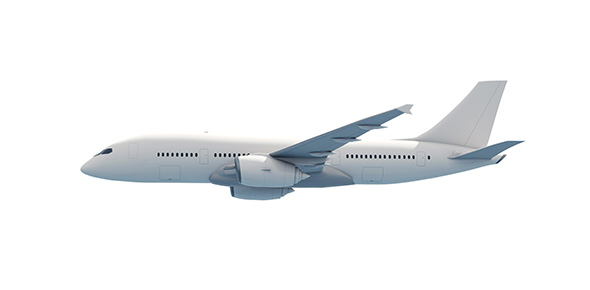# Avionics Fundamentals - Vor, Rmi, DME

15 Questions | Attempts: 1992
ShareSettings15 questions based on the FSS Web-X presentation,� Introduction to Avionic Fundamentals 4 - VOR, RMI, DME � �

• 1.
VOR generates two signals on the carrier frequency?
• A.

True

• B.

False

• 2.
The Reference and Variable signals are in phase at 180deg magnetic?
• A.

True

• B.

False

• 3.
The VOR radials are outbound from a station?
• A.

True

• B.

False

• 4.
What is the Variable Frequency of VOR?
• A.

30Hz

• B.

60Hz

• C.

1020Hz

• D.

9960Hz

• 5.
What is the sub-carrier Reference Frequency of VOR?
• A.

1020Hz

• B.

108.5Mhz

• C.

9960Hz

• 6.
How is the Variable Frequency of VOR modulated?
• A.

Frequency Modulated

• B.

Phase Modulated

• C.

Amplitude Modulated

• 7.
RMI comapss information is referred as?
• A.

Bearing needle

• B.

Vertical gyro

• C.

Horizontal gyro

• D.

Slaved compass

• 8.
The Standby compass indicates True North?
• A.

True

• B.

False

• 9.
The pointers on the RMI indicate?
• A.

• B.

Bearing

• C.

• 10.
DME is usually used in conjuction with:
• A.

• B.

ILS

• C.

VOR

• 11.
DME supplies range information up to how many miles?
• A.

50 NM

• B.

125 NM

• C.

250 NM

• 12.
The VOR frequency range is 9510 Hz to 10410 Hz
• A.

True

• B.

False

• 13.
What is 'Slant Range'?
• A.

Same as Ground Distance

• B.

Actual line-of-sight distance to the station

• C.

Range to the horizon in NM

• 14.
What is the DME frequency range?
• A.

960-1215 Mhz

• B.

108-112 Mhz

• C.

200-1700 Mhz

• 15.
An HSI uses what type of gyroscope?
• A.

Vertical Gyro

• B.

Horizontal Gyro

• C.

Standby Gyro

## Related TopicsBack to top
×

Wait!
Here's an interesting quiz for you.